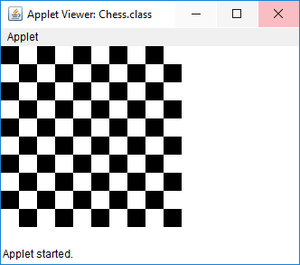GFG App
Open AppBrowser
Continue

Given task is to draw a Chessboard in Java Applet

Approach:

1. Create a rectangle with length and breadth of 20 unit each, with 10 rows and columns of chess.
2. As soon as even position occurs in row and column change the color of a rectangle with BLACK, else it will be WHITE

Below is the implementation of the above approach:

Applet Program:

## Java

 `import` `java.applet.*;` `import` `java.awt.*;` `/*` `*/` `// Extends Applet Class` `public` `class` `Chess ``extends` `Applet {`   `    ``static` `int` `N = ``10``;`   `    ``// Use paint() method` `    ``public` `void` `paint(Graphics g)` `    ``{` `        ``int` `x, y;` `        ``for` `(``int` `row = ``0``; row & lt; N; row++) {`   `            ``for` `(``int` `col = ``0``; col & lt; N; col++) {`   `                ``// Set x coordinates of rectangle` `                ``// by 20 times` `                ``x = row * ``20``;`   `                ``// Set y coordinates of rectangle` `                ``// by 20 times` `                ``y = col * ``20``;`   `                ``// Check whether row and column` `                ``// are in even position` `                ``// If it is true set Black color` `                ``if` `((row % ``2` `== ``0``) == (col % ``2` `== ``0``))` `                    ``g.setColor(Color.BLACK);` `                ``else` `                    ``g.setColor(Color.WHITE);`   `                ``// Create a rectangle with` `                ``// length and breadth of 20` `                ``g.fillRect(x, y, ``20``, ``20``);` `            ``}` `        ``}` `    ``}` `}`

Output:Note: To run the applet in command line use the following commands.

```> javac Chess.java
> appletviewer Chess.java```

You can also refer to: https://www.geeksforgeeks.org/different-ways-to-run-applet-in-java to run applet program.

My Personal Notes arrow_drop_up